# Inverse Kinematics VI

LabVIEW 2014 Robotics Module Help

Edition Date: June 2014

Part Number: 372983F-01

»View Product InfoDownload Help (Windows Only)

Owning Palette: Kinematics VIs

Requires: Robotics Module

Computes the joint angles of a robotic arm given the location of the end effector relative to the arm base. Wire data to the end effector transform input to determine the polymorphic instance to use or manually select the instance.

.m file: ikine

This VI uses a numerical solver, while the Analytical Inverse Kinematics VI provides an analytical solver. Choose an inverse kinematics solver according to the arm type you generate.

Example

Use the pull-down menu to select an instance of this VI.

 Select an instance Inverse Kinematics (Point)Inverse Kinematics (Trajectory)

## Inverse Kinematics (Point)

This instance calculates joint positions for a single end effector position.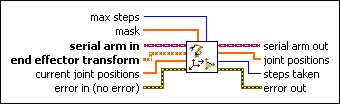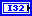max steps specifies the greatest number of times the inverse kinematics solver runs. The default is 100. As max steps increases, so does the accuracy of the joint positions this VI calculates. LabVIEW returns an error if this VI cannot converge to a solution within max steps.mask is a six-element array of values that scale each iteration of the inverse kinematics solver. mask is a 1D array of six elements in the form of [x, y, z, theta_x, theta_y, theta_z], where x, y, z correspond to translation along the x-, y-, and z-axes and theta_x, theta_y, theta_z correspond to rotation about the x-, y-, and z-axes, respectively. If one of these elements is unconstrained, for example, because the arm does not have enough degrees of freedom to control the element, specify 0 for the corresponding element of mask to allow this VI to reach a solution except for the masked element. By default, mask is [1, 1, 1, 1, 1, 1].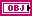serial arm in is a reference to a serial robotics arm. Use the Initialize Serial Arm VI to generate this LabVIEW class object.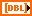end effector transform specifies the homogenous transform that represents the position of the end effector relative to the arm base.current joint positions specifies a guess of the joint angles, in radians, that make up the joint positions in the inverse kinematics solution.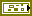error in describes error conditions that occur before this node runs. This input provides standard error in functionality.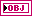serial arm out is a reference to a serial robotic arm. You can wire this output to other Robotic Arm VIs.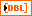joint positions contains the calculated joint angles of the robotic arm pose given the position of the end effector.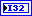steps taken returns the number of iterations the inverse kinematics solver performs to calculate joint position.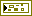error out contains error information. This output provides standard error out functionality.

## Inverse Kinematics (Trajectory)

This instance calculates joint positions given end effector positions at points along a trajectory. Use the Generate Cartesian Trajectory VI to calculate end effector positions along a trajectory you can pass to this instance to calculate inverse kinematics for the joint positions.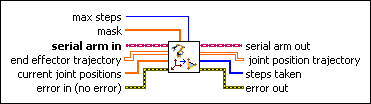max steps specifies the greatest number of times the inverse kinematics solver runs. The default is 100. As max steps increases, so does the accuracy of the joint positions this VI calculates. LabVIEW returns an error if this VI cannot converge to a solution within max steps.mask is a six-element array of values that scale each iteration of the inverse kinematics solver. mask is a 1D array of six elements in the form of [x, y, z, theta_x, theta_y, theta_z], where x, y, z correspond to translation along the x-, y-, and z-axes and theta_x, theta_y, theta_z correspond to rotation about the x-, y-, and z-axes, respectively. If one of these elements is unconstrained, for example, because the arm does not have enough degrees of freedom to control the element, specify 0 for the corresponding element of mask to allow this VI to reach a solution except for the masked element. By default, mask is [1, 1, 1, 1, 1, 1].serial arm in is a reference to a serial robotics arm. Use the Initialize Serial Arm VI to generate this LabVIEW class object.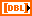end effector trajectory specifies the homogenous transforms that represent the position of the end effector at points along a trajectory.current joint positions specifies a guess of the joint angles, in radians, that make up the joint positions in the inverse kinematics solution.error in describes error conditions that occur before this node runs. This input provides standard error in functionality.serial arm out is a reference to a serial robotic arm. You can wire this output to other Robotic Arm VIs.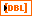joint position trajectory contains the calculated joint angles of the robotic arm pose where each row represents a pose at a particular time and each column represents a particular joint.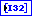steps taken returns the number of iterations the inverse kinematics solver performs to calculate the solution at each point along joint position trajectory.error out contains error information. This output provides standard error out functionality.

## Example

Refer to the Robot Arm Examples.lvproj in the labview\examples\robotics\Robotic Arm directory for an example of using the Inverse Kinematics VI.